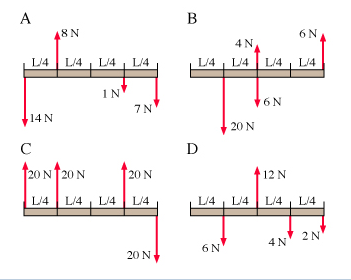# Problem: Consider the figure. For which diagrams is the angular momentum constant?

###### FREE Expert Solution

The angular momentum is constant if the net torque is zero.

100% (226 ratings)###### Problem Details

Consider the figure. For which diagrams is the angular momentum constant?